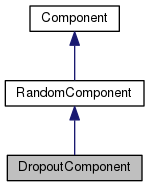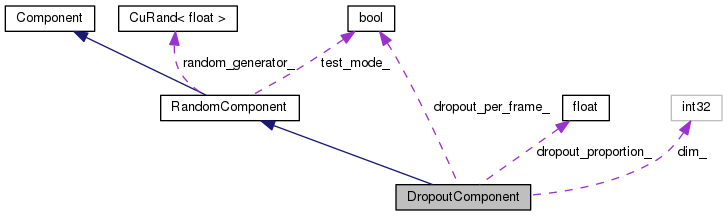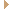DropoutComponent Class Reference

`#include <nnet-simple-component.h>`

Inheritance diagram for DropoutComponent:[legend]
Collaboration diagram for DropoutComponent:[legend]

## Public Member Functions

void Init (int32 dim, BaseFloat dropout_proportion=0.0, bool dropout_per_frame=false)

DropoutComponent (int32 dim, BaseFloat dropout=0.0, bool dropout_per_frame=false)

DropoutComponent ()

DropoutComponent (const DropoutComponent &other)

virtual int32 Properties () const
Return bitmask of the component's properties. More...

virtual std::string Type () const
Returns a string such as "SigmoidComponent", describing the type of the object. More...

virtual void InitFromConfig (ConfigLine *cfl)
Initialize, from a ConfigLine object. More...

virtual int32 InputDim () const
Returns input-dimension of this component. More...

virtual int32 OutputDim () const
Returns output-dimension of this component. More...

virtual void Read (std::istream &is, bool binary)
Read function (used after we know the type of the Component); accepts input that is missing the token that describes the component type, in case it has already been consumed. More...

virtual void Write (std::ostream &os, bool binary) const
Write component to stream. More...

virtual void * Propagate (const ComponentPrecomputedIndexes *indexes, const CuMatrixBase< BaseFloat > &in, CuMatrixBase< BaseFloat > *out) const
Propagate function. More...

virtual void Backprop (const std::string &debug_info, const ComponentPrecomputedIndexes *indexes, const CuMatrixBase< BaseFloat > &in_value, const CuMatrixBase< BaseFloat > &out_value, const CuMatrixBase< BaseFloat > &out_deriv, void *memo, Component *to_update, CuMatrixBase< BaseFloat > *in_deriv) const
Backprop function; depending on which of the arguments 'to_update' and 'in_deriv' are non-NULL, this can compute input-data derivatives and/or perform model update. More...

virtual ComponentCopy () const
Copies component (deep copy). More...

virtual std::string Info () const

void SetDropoutProportion (BaseFloat dropout_proportion)

BaseFloat DropoutProportion () constPublic Member Functions inherited from RandomComponent
void ResetGenerator ()

void SetTestMode (bool test_mode)

RandomComponent ()

RandomComponent (const RandomComponent &other)Public Member Functions inherited from Component
virtual void StoreStats (const CuMatrixBase< BaseFloat > &in_value, const CuMatrixBase< BaseFloat > &out_value, void *memo)
This function may store stats on average activation values, and for some component types, the average value of the derivative of the nonlinearity. More...

virtual void ZeroStats ()
Components that provide an implementation of StoreStats should also provide an implementation of ZeroStats(), to set those stats to zero. More...

virtual void GetInputIndexes (const MiscComputationInfo &misc_info, const Index &output_index, std::vector< Index > *desired_indexes) const
This function only does something interesting for non-simple Components. More...

virtual bool IsComputable (const MiscComputationInfo &misc_info, const Index &output_index, const IndexSet &input_index_set, std::vector< Index > *used_inputs) const
This function only does something interesting for non-simple Components, and it exists to make it possible to manage optionally-required inputs. More...

virtual void ReorderIndexes (std::vector< Index > *input_indexes, std::vector< Index > *output_indexes) const
This function only does something interesting for non-simple Components. More...

virtual ComponentPrecomputedIndexesPrecomputeIndexes (const MiscComputationInfo &misc_info, const std::vector< Index > &input_indexes, const std::vector< Index > &output_indexes, bool need_backprop) const
This function must return NULL for simple Components. More...

virtual void Scale (BaseFloat scale)
This virtual function when called on – an UpdatableComponent scales the parameters by "scale" when called by an UpdatableComponent. More...

virtual void Add (BaseFloat alpha, const Component &other)
This virtual function when called by – an UpdatableComponent adds the parameters of another updatable component, times some constant, to the current parameters. More...

virtual void DeleteMemo (void *memo) const
This virtual function only needs to be overwritten by Components that return a non-NULL memo from their Propagate() function. More...

virtual void ConsolidateMemory ()
This virtual function relates to memory management, and avoiding fragmentation. More...

Component ()

virtual ~Component ()

## Private Attributes

int32 dim_

BaseFloat dropout_proportion_
dropout-proportion is the proportion that is dropped out, e.g. More...

bool dropout_per_frame_Static Public Member Functions inherited from Component
static ComponentReadNew (std::istream &is, bool binary)
Read component from stream (works out its type). Dies on error. More...

static ComponentNewComponentOfType (const std::string &type)
Returns a new Component of the given type e.g. More...Protected Attributes inherited from RandomComponent
CuRand< BaseFloatrandom_generator_

bool test_mode_

## Detailed Description

Definition at line 92 of file nnet-simple-component.h.

## ◆ DropoutComponent() [1/3]

 DropoutComponent ( int32 dim, BaseFloat dropout = `0.0`, bool dropout_per_frame = `false` )
inline

Definition at line 97 of file nnet-simple-component.h.

References PnormComponent::Init().

98  {
99  Init(dim, dropout, dropout_per_frame);
100  }
void Init(int32 dim, BaseFloat dropout_proportion=0.0, bool dropout_per_frame=false)

## ◆ DropoutComponent() [2/3]

 DropoutComponent ( )
inline

Definition at line 102 of file nnet-simple-component.h.

Referenced by DropoutComponent::Copy().

102  : dim_(0), dropout_proportion_(0.0),
103  dropout_per_frame_(false) { }
BaseFloat dropout_proportion_
dropout-proportion is the proportion that is dropped out, e.g.

## ◆ DropoutComponent() [3/3]

 DropoutComponent ( const DropoutComponent & other )

Definition at line 94 of file nnet-simple-component.cc.

94  :
95  RandomComponent(other),
96  dim_(other.dim_),
97  dropout_proportion_(other.dropout_proportion_),
98  dropout_per_frame_(other.dropout_per_frame_) { }
BaseFloat dropout_proportion_
dropout-proportion is the proportion that is dropped out, e.g.

## ◆ Backprop()

 void Backprop ( const std::string & debug_info, const ComponentPrecomputedIndexes * indexes, const CuMatrixBase< BaseFloat > & in_value, const CuMatrixBase< BaseFloat > & out_value, const CuMatrixBase< BaseFloat > & out_deriv, void * memo, Component * to_update, CuMatrixBase< BaseFloat > * in_deriv ) const
virtual

Backprop function; depending on which of the arguments 'to_update' and 'in_deriv' are non-NULL, this can compute input-data derivatives and/or perform model update.

Parameters
 [in] debug_info The component name, to be printed out in any warning messages. [in] indexes A pointer to some information output by this class's PrecomputeIndexes function (will be NULL for simple components, i.e. those that don't do things like splicing). [in] in_value The matrix that was given as input to the Propagate function. Will be ignored (and may be empty) if Properties()&kBackpropNeedsInput == 0. [in] out_value The matrix that was output from the Propagate function. Will be ignored (and may be empty) if Properties()&kBackpropNeedsOutput == 0 [in] out_deriv The derivative at the output of this component. [in] memo This will normally be NULL, but for component types that set the flag kUsesMemo, this will be the return value of the Propagate() function that corresponds to this Backprop() function. Ownership of any pointers is not transferred to the Backprop function; DeleteMemo() will be called to delete it. [out] to_update If model update is desired, the Component to be updated, else NULL. Does not have to be identical to this. If supplied, you can assume that to_update->Properties() & kUpdatableComponent is nonzero. [out] in_deriv The derivative at the input of this component, if needed (else NULL). If Properties()&kBackpropInPlace, may be the same matrix as out_deriv. If Properties()&kBackpropAdds, this is added to by the Backprop routine, else it is set. The component code chooses which mode to work in, based on convenience.

Implements Component.

Definition at line 179 of file nnet-simple-component.cc.

186  {
187  NVTX_RANGE("DropoutComponent::Backprop");
188  KALDI_ASSERT(in_value.NumRows() == out_value.NumRows() &&
189  in_value.NumCols() == out_value.NumCols());
190
191  KALDI_ASSERT(in_value.NumRows() == out_deriv.NumRows() &&
192  in_value.NumCols() == out_deriv.NumCols());
193  in_deriv->SetMatMatDivMat(out_deriv, out_value, in_value);
194 }
#define KALDI_ASSERT(cond)
Definition: kaldi-error.h:185
#define NVTX_RANGE(name)
Definition: cu-common.h:143

## ◆ Copy()

 Component * Copy ( ) const
virtual

Copies component (deep copy).

Implements Component.

Definition at line 100 of file nnet-simple-component.cc.

References DropoutComponent::DropoutComponent().

100  {
101  DropoutComponent *ans = new DropoutComponent(*this);
102  return ans;
103 }

## ◆ DropoutProportion()

 BaseFloat DropoutProportion ( ) const
inline

Definition at line 144 of file nnet-simple-component.h.

Referenced by ModelCollapser::CollapseComponentsDropout().

144 { return dropout_proportion_; }
BaseFloat dropout_proportion_
dropout-proportion is the proportion that is dropped out, e.g.

## ◆ Info()

 std::string Info ( ) const
virtual

Starts with the type of the component. E.g. "SigmoidComponent dim=900", although most components will have much more info.

Reimplemented from Component.

Definition at line 131 of file nnet-simple-component.cc.

131  {
132  std::ostringstream stream;
133  stream << Type() << ", dim=" << dim_
134  << ", dropout-proportion=" << dropout_proportion_
135  << ", dropout-per-frame=" << (dropout_per_frame_ ? "true" : "false");
136  return stream.str();
137 }
virtual std::string Type() const
Returns a string such as "SigmoidComponent", describing the type of the object.
BaseFloat dropout_proportion_
dropout-proportion is the proportion that is dropped out, e.g.

## ◆ Init()

 void Init ( int32 dim, BaseFloat dropout_proportion = `0.0`, bool dropout_per_frame = `false` )

Definition at line 105 of file nnet-simple-component.cc.

106  {
107  dropout_proportion_ = dropout_proportion;
108  dropout_per_frame_ = dropout_per_frame;
109  dim_ = dim;
110 }
BaseFloat dropout_proportion_
dropout-proportion is the proportion that is dropped out, e.g.

## ◆ InitFromConfig()

 void InitFromConfig ( ConfigLine * cfl )
virtual

Initialize, from a ConfigLine object.

Parameters
 [in] cfl A ConfigLine containing any parameters that are needed for initialization. For example: "dim=100 param-stddev=0.1"

Implements Component.

Definition at line 112 of file nnet-simple-component.cc.

112  {
113  int32 dim = 0;
114  BaseFloat dropout_proportion = 0.0;
115  bool dropout_per_frame = false;
116  test_mode_ = false;
117  bool ok = cfl->GetValue("dim", &dim) &&
118  cfl->GetValue("dropout-proportion", &dropout_proportion);
119  cfl->GetValue("dropout-per-frame", &dropout_per_frame);
120  // It only makes sense to set test-mode in the config for testing purposes.
121  cfl->GetValue("test-mode", &test_mode_);
122  // for this stage, dropout is hard coded in
123  // normal mode if not declared in config
124  if (!ok || cfl->HasUnusedValues() || dim <= 0 ||
125  dropout_proportion < 0.0 || dropout_proportion > 1.0)
126  KALDI_ERR << "Invalid initializer for layer of type "
127  << Type() << ": \"" << cfl->WholeLine() << "\"";
128  Init(dim, dropout_proportion, dropout_per_frame);
129 }
kaldi::int32 int32
float BaseFloat
Definition: kaldi-types.h:29
virtual std::string Type() const
Returns a string such as "SigmoidComponent", describing the type of the object.
#define KALDI_ERR
Definition: kaldi-error.h:147
void Init(int32 dim, BaseFloat dropout_proportion=0.0, bool dropout_per_frame=false)

## ◆ InputDim()

 virtual int32 InputDim ( ) const
inlinevirtual

Returns input-dimension of this component.

Implements Component.

Definition at line 115 of file nnet-simple-component.h.

## ◆ OutputDim()

 virtual int32 OutputDim ( ) const
inlinevirtual

Returns output-dimension of this component.

Implements Component.

Definition at line 117 of file nnet-simple-component.h.

## ◆ Propagate()

 void * Propagate ( const ComponentPrecomputedIndexes * indexes, const CuMatrixBase< BaseFloat > & in, CuMatrixBase< BaseFloat > * out ) const
virtual

Propagate function.

Parameters
 [in] indexes A pointer to some information output by this class's PrecomputeIndexes function (will be NULL for simple components, i.e. those that don't do things like splicing). [in] in The input to this component. Num-columns == InputDim(). [out] out The output of this component. Num-columns == OutputDim(). Note: output of this component will be added to the initial value of "out" if Properties()&kPropagateAdds != 0; otherwise the output will be set and the initial value ignored. Each Component chooses whether it is more convenient implementation-wise to add or set, and the calling code has to deal with it.
Returns
Normally returns NULL, but may return a non-NULL value for components which have the flag kUsesMemo set. This value will be passed into the corresponding Backprop routine.

Implements Component.

Definition at line 139 of file nnet-simple-component.cc.

141  {
142  KALDI_ASSERT(out->NumRows() == in.NumRows() && out->NumCols() == in.NumCols()
143  && in.NumCols() == dim_);
144
145  BaseFloat dropout = dropout_proportion_;
146  KALDI_ASSERT(dropout >= 0.0 && dropout <= 1.0);
147  if (test_mode_) {
148  out->CopyFromMat(in);
149  out->Scale(1.0 - dropout);
150  return NULL;
151  }
152  if (!dropout_per_frame_) {
153  // This const_cast is only safe assuming you don't attempt
154  // to use multi-threaded code with the GPU.
155  const_cast<CuRand<BaseFloat>&>(random_generator_).RandUniform(out);
156
157  out->Add(-dropout); // now, a proportion "dropout" will be <0.0
158  // apply the function (x>0?1:0). Now, a proportion
159  // "dropout" will be zero and (1 - dropout) will be 1.0.
160  out->ApplyHeaviside();
161
162  out->MulElements(in);
163  } else {
164  // randomize the dropout matrix by row,
165  // i.e. [[1,1,1,1],[0,0,0,0],[0,0,0,0],[1,1,1,1],[0,0,0,0]]
166  CuMatrix<BaseFloat> tmp(1, out->NumRows(), kUndefined);
167  // This const_cast is only safe assuming you don't attempt
168  // to use multi-threaded code with the GPU.
169  const_cast<CuRand<BaseFloat>&>(random_generator_).RandUniform(&tmp);
171  tmp.ApplyHeaviside();
172  out->CopyColsFromVec(tmp.Row(0));
173  out->MulElements(in);
174  }
175  return NULL;
176 }
float RandUniform(struct RandomState *state=NULL)
Returns a random number strictly between 0 and 1.
Definition: kaldi-math.h:151
float BaseFloat
Definition: kaldi-types.h:29
#define KALDI_ASSERT(cond)
Definition: kaldi-error.h:185
CuRand< BaseFloat > random_generator_
BaseFloat dropout_proportion_
dropout-proportion is the proportion that is dropped out, e.g.

## ◆ Properties()

 virtual int32 Properties ( ) const
inlinevirtual

Return bitmask of the component's properties.

These properties depend only on the component's type. See enum ComponentProperties.

Implements Component.

Definition at line 107 of file nnet-simple-component.h.

107  {
110  }

 void Read ( std::istream & is, bool binary )
virtual

Read function (used after we know the type of the Component); accepts input that is missing the token that describes the component type, in case it has already been consumed.

Implements Component.

Definition at line 198 of file nnet-simple-component.cc.

198  {
199  std::string token;
201  if (token == "<DropoutComponent>") {
203  }
204  KALDI_ASSERT(token == "<Dim>");
207  KALDI_ASSERT(token == "<DropoutProportion>");
210  if (token == "<DropoutPerFrame>") {
213  } else {
214  dropout_per_frame_ = false;
215  }
216  if (token == "<TestMode>") {
218  ExpectToken(is, binary, "</DropoutComponent>");
219  } else {
220  test_mode_ = false;
221  KALDI_ASSERT(token == "</DropoutComponent>");
222  }
223 }
void ReadBasicType(std::istream &is, bool binary, T *t)
ReadBasicType is the name of the read function for bool, integer types, and floating-point types...
Definition: io-funcs-inl.h:55
void ReadToken(std::istream &is, bool binary, std::string *str)
ReadToken gets the next token and puts it in str (exception on failure).
Definition: io-funcs.cc:154
static void ExpectToken(const std::string &token, const std::string &what_we_are_parsing, const std::string **next_token)
#define KALDI_ASSERT(cond)
Definition: kaldi-error.h:185
BaseFloat dropout_proportion_
dropout-proportion is the proportion that is dropped out, e.g.

## ◆ SetDropoutProportion()

 void SetDropoutProportion ( BaseFloat dropout_proportion )
inline

Definition at line 140 of file nnet-simple-component.h.

140  {
141  dropout_proportion_ = dropout_proportion;
142  }
BaseFloat dropout_proportion_
dropout-proportion is the proportion that is dropped out, e.g.

## ◆ Type()

 virtual std::string Type ( ) const
inlinevirtual

Returns a string such as "SigmoidComponent", describing the type of the object.

Implements Component.

Definition at line 111 of file nnet-simple-component.h.

References PnormComponent::InitFromConfig().

111 { return "DropoutComponent"; }

## ◆ Write()

 void Write ( std::ostream & os, bool binary ) const
virtual

Write component to stream.

Implements Component.

Definition at line 225 of file nnet-simple-component.cc.

225  {
226  WriteToken(os, binary, "<DropoutComponent>");
227  WriteToken(os, binary, "<Dim>");
228  WriteBasicType(os, binary, dim_);
229  WriteToken(os, binary, "<DropoutProportion>");
230  WriteBasicType(os, binary, dropout_proportion_);
231  WriteToken(os, binary, "<DropoutPerFrame>");
232  WriteBasicType(os, binary, dropout_per_frame_);
233  WriteToken(os, binary, "<TestMode>");
234  WriteBasicType(os, binary, test_mode_);
235  WriteToken(os, binary, "</DropoutComponent>");
236 }
void WriteToken(std::ostream &os, bool binary, const char *token)
The WriteToken functions are for writing nonempty sequences of non-space characters.
Definition: io-funcs.cc:134
void WriteBasicType(std::ostream &os, bool binary, T t)
WriteBasicType is the name of the write function for bool, integer types, and floating-point types...
Definition: io-funcs-inl.h:34
BaseFloat dropout_proportion_
dropout-proportion is the proportion that is dropped out, e.g.

## ◆ dropout_per_frame_

 bool dropout_per_frame_
private

Definition at line 150 of file nnet-simple-component.h.

## ◆ dropout_proportion_

 BaseFloat dropout_proportion_
private

dropout-proportion is the proportion that is dropped out, e.g.

if 0.1, we set 10% to zero value.

Definition at line 149 of file nnet-simple-component.h.

The documentation for this class was generated from the following files: